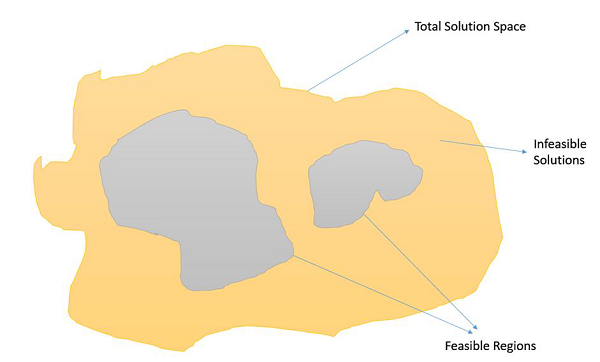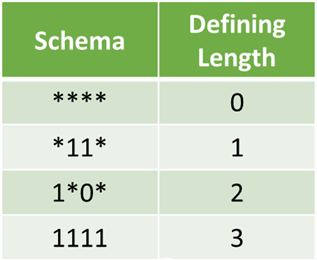# Genetic Algorithms - Advanced Topics

In this section, we introduce some advanced topics in Genetic Algorithms. A reader looking for just an introduction to GAs may choose to skip this section.

## Constrained Optimization Problems

Constrained Optimization Problems are those optimization problems in which we have to maximize or minimize a given objective function value that is subject to certain constraints. Therefore, not all results in the solution space are feasible, and the solution space contains feasible regions as shown in the following image.In such a scenario, crossover and mutation operators might give us solutions which are infeasible. Therefore, additional mechanisms have to be employed in the GA when dealing with constrained Optimization Problems.

Some of the most common methods are −

• Using penalty functions which reduces the fitness of infeasible solutions, preferably so that the fitness is reduced in proportion with the number of constraints violated or the distance from the feasible region.

• Using repair functions which take an infeasible solution and modify it so that the violated constraints get satisfied.

• Not allowing infeasible solutions to enter into the population at all.

• Use a special representation or decoder functions that ensures feasibility of the solutions.

## Basic Theoretical Background

In this section, we will discuss about the Schema and NFL theorem along with the building block hypothesis.

### Schema Theorem

Researchers have been trying to figure out the mathematics behind the working of genetic algorithms, and Holland’s Schema Theorem is a step in that direction. Over the year’s various improvements and suggestions have been done to the Schema Theorem to make it more general.

In this section, we don’t delve into the mathematics of the Schema Theorem, rather we try to develop a basic understanding of what the Schema Theorem is. The basic terminology to know are as follows −

• A Schema is a “template”. Formally, it is a string over the alphabet = {0,1,*},

where * is don’t care and can take any value.

Therefore, *10*1 could mean 01001, 01011, 11001, or 11011

Geometrically, a schema is a hyper-plane in the solution search space.

• Order of a schema is the number of specified fixed positions in a gene.• Defining length is the distance between the two furthest fixed symbols in the gene.The schema theorem states that this schema with above average fitness, short defining length and lower order is more likely to survive crossover and mutation.

### Building Block Hypothesis

Building Blocks are low order, low defining length schemata with the above given average fitness. The building block hypothesis says that such building blocks serve as a foundation for the GAs success and adaptation in GAs as it progresses by successively identifying and recombining such “building blocks”.

### No Free Lunch (NFL) Theorem

Wolpert and Macready in 1997 published a paper titled "No Free Lunch Theorems for Optimization." It essentially states that if we average over the space of all possible problems, then all non-revisiting black box algorithms will exhibit the same performance.

It means that the more we understand a problem, our GA becomes more problem specific and gives better performance, but it makes up for that by performing poorly for other problems.

## GA Based Machine Learning

Genetic Algorithms also find application in Machine Learning. Classifier systems are a form of genetics-based machine learning (GBML) system that are frequently used in the field of machine learning. GBML methods are a niche approach to machine learning.

There are two categories of GBML systems −

• The Pittsburg Approach − In this approach, one chromosome encoded one solution, and so fitness is assigned to solutions.

• The Michigan Approach − one solution is typically represented by many chromosomes and so fitness is assigned to partial solutions.

It should be kept in mind that the standard issue like crossover, mutation, Lamarckian or Darwinian, etc. are also present in the GBML systems.